The Axioms of Geometry (1899)* By Bertrand Russell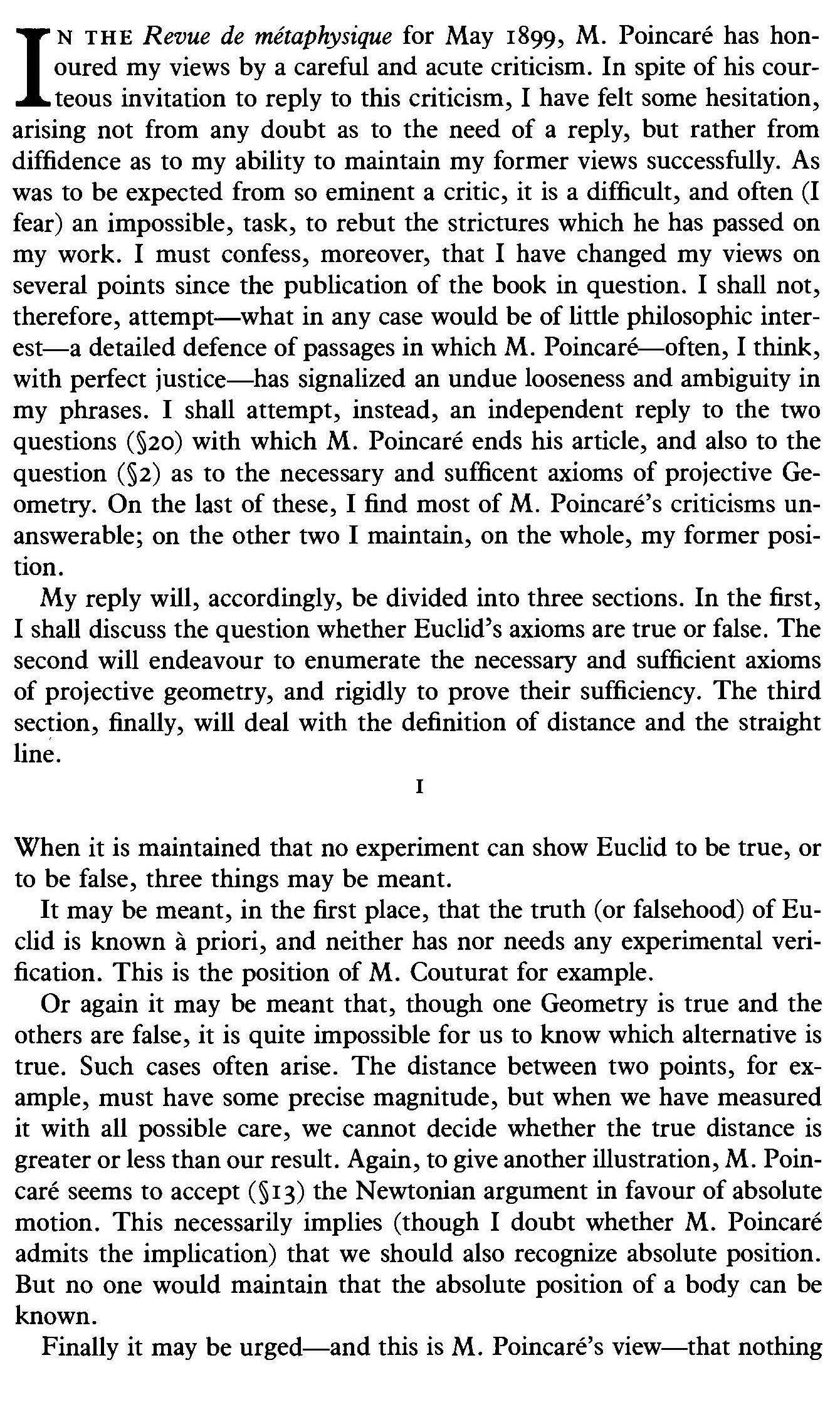The Axioms of Geometry 2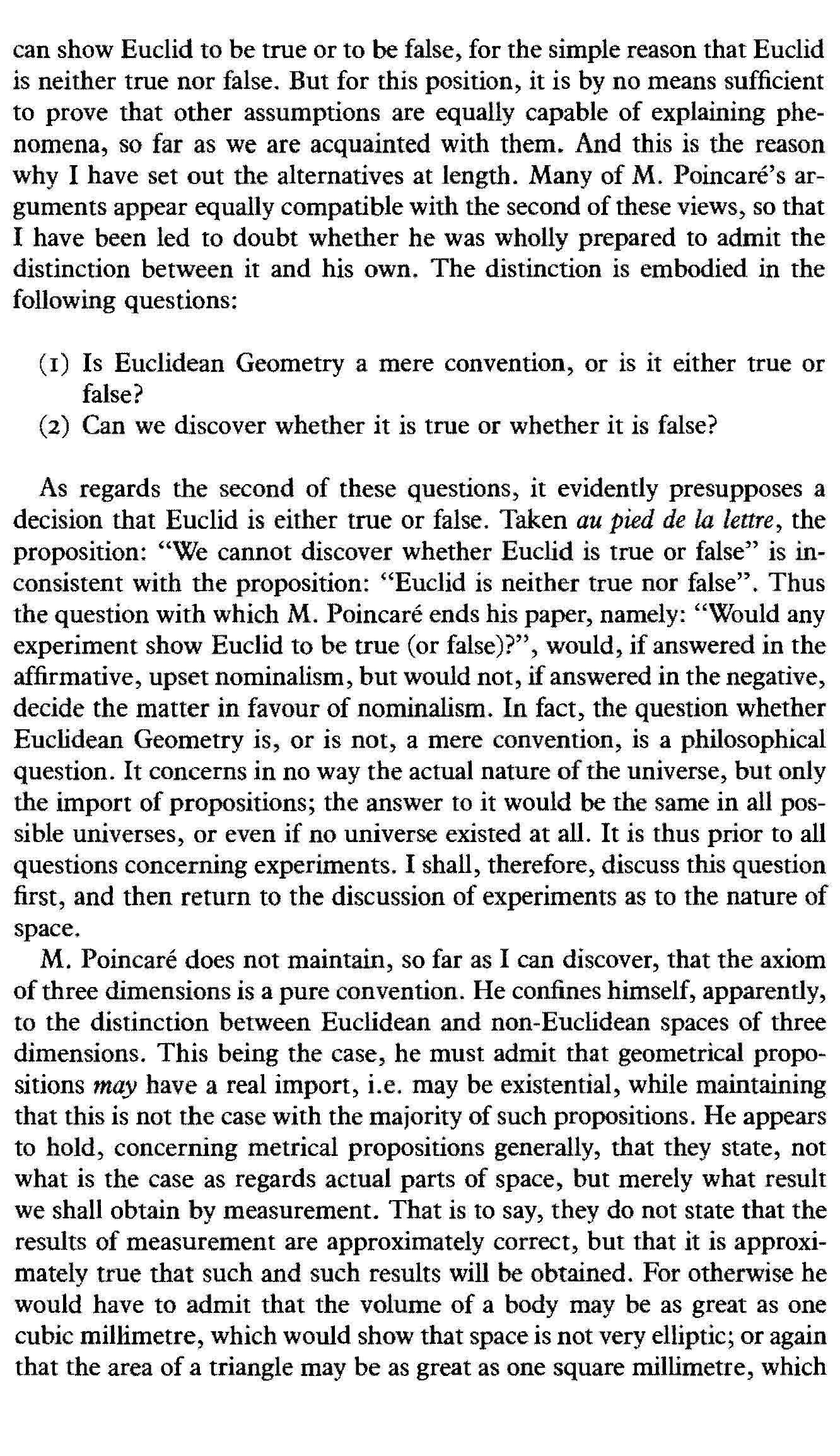The Axioms of Geometry 3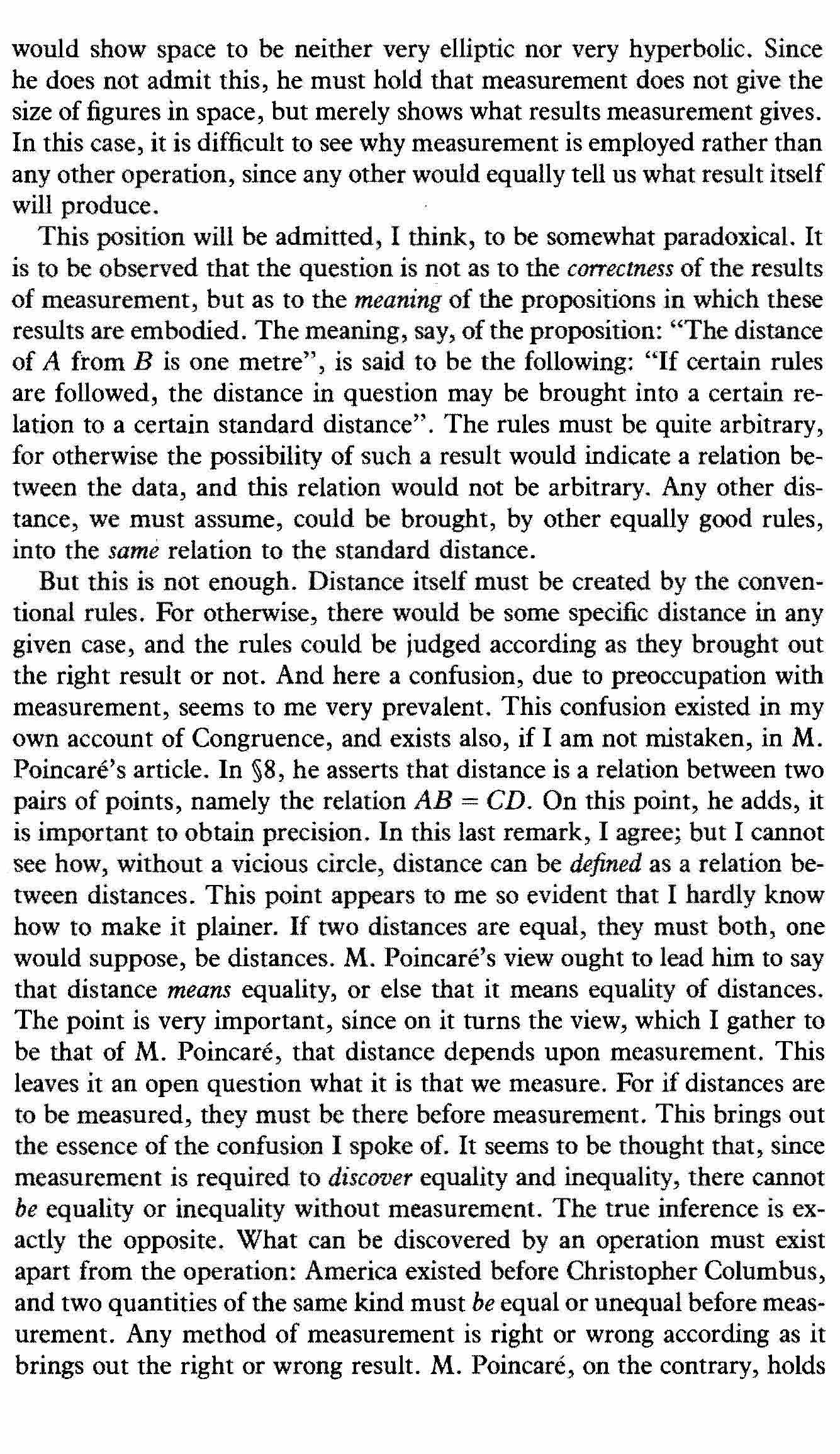The Axioms of Geometry 4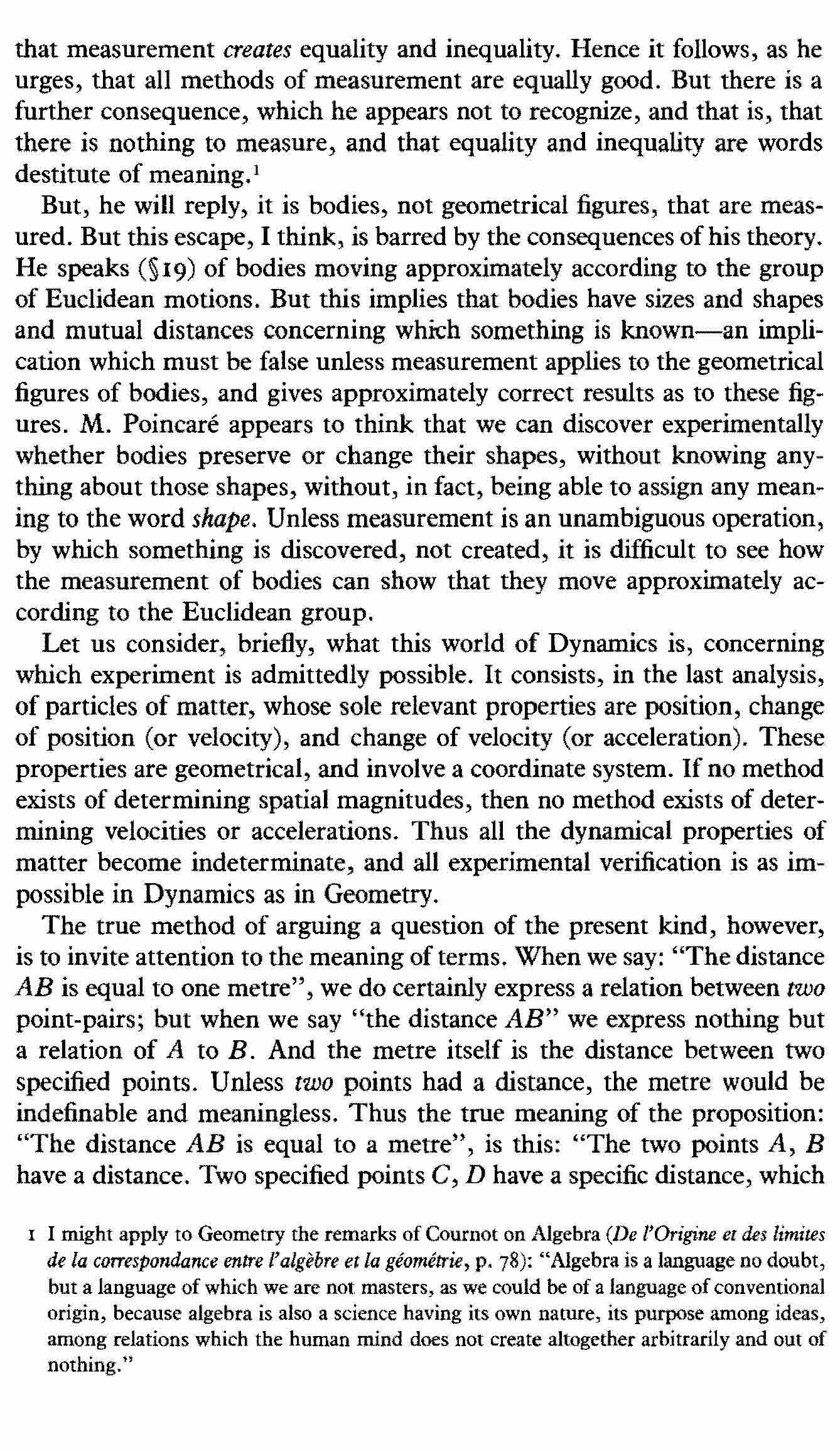The Axioms of Geometry 5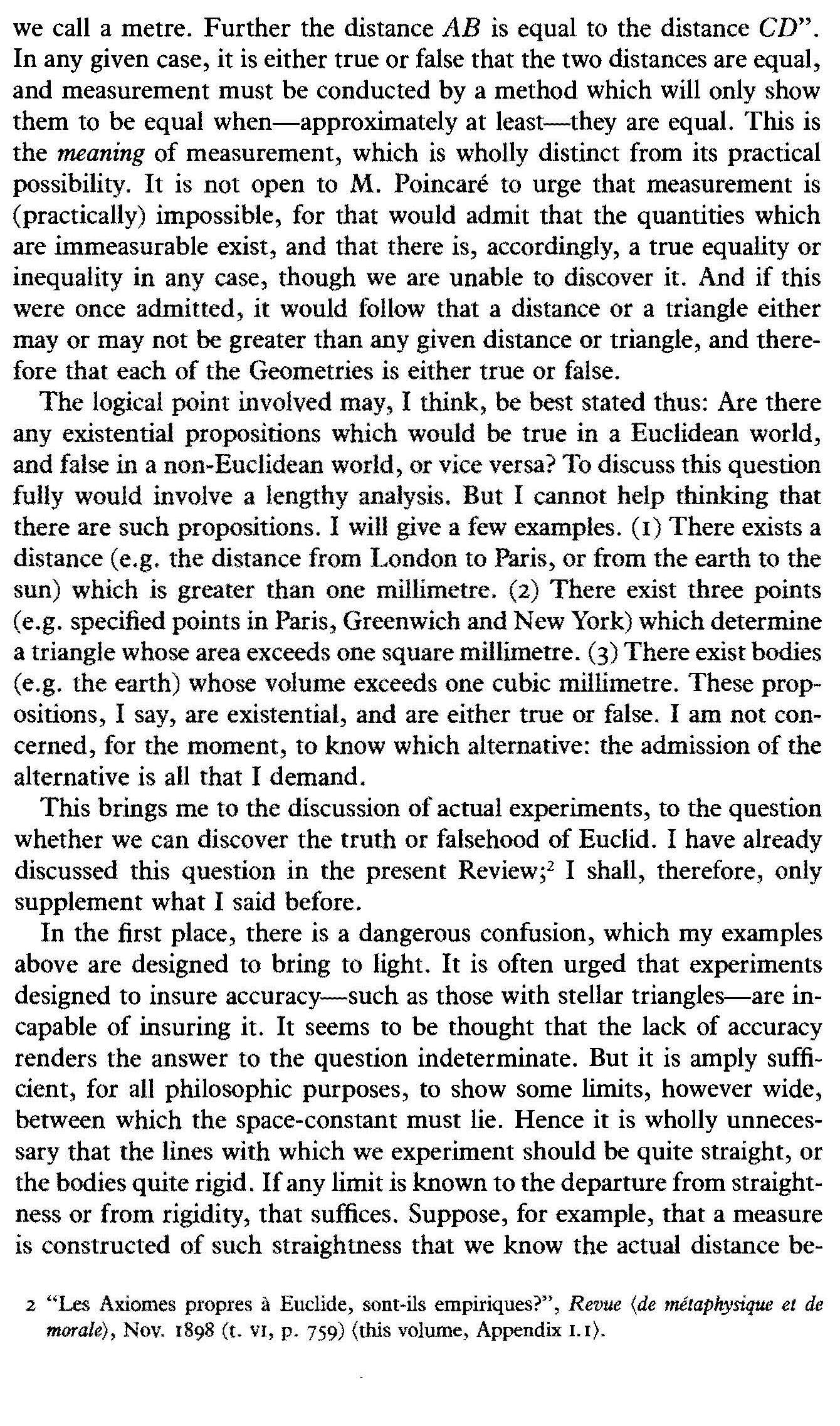The Axioms of Geometry 6The Axioms of Geometry 7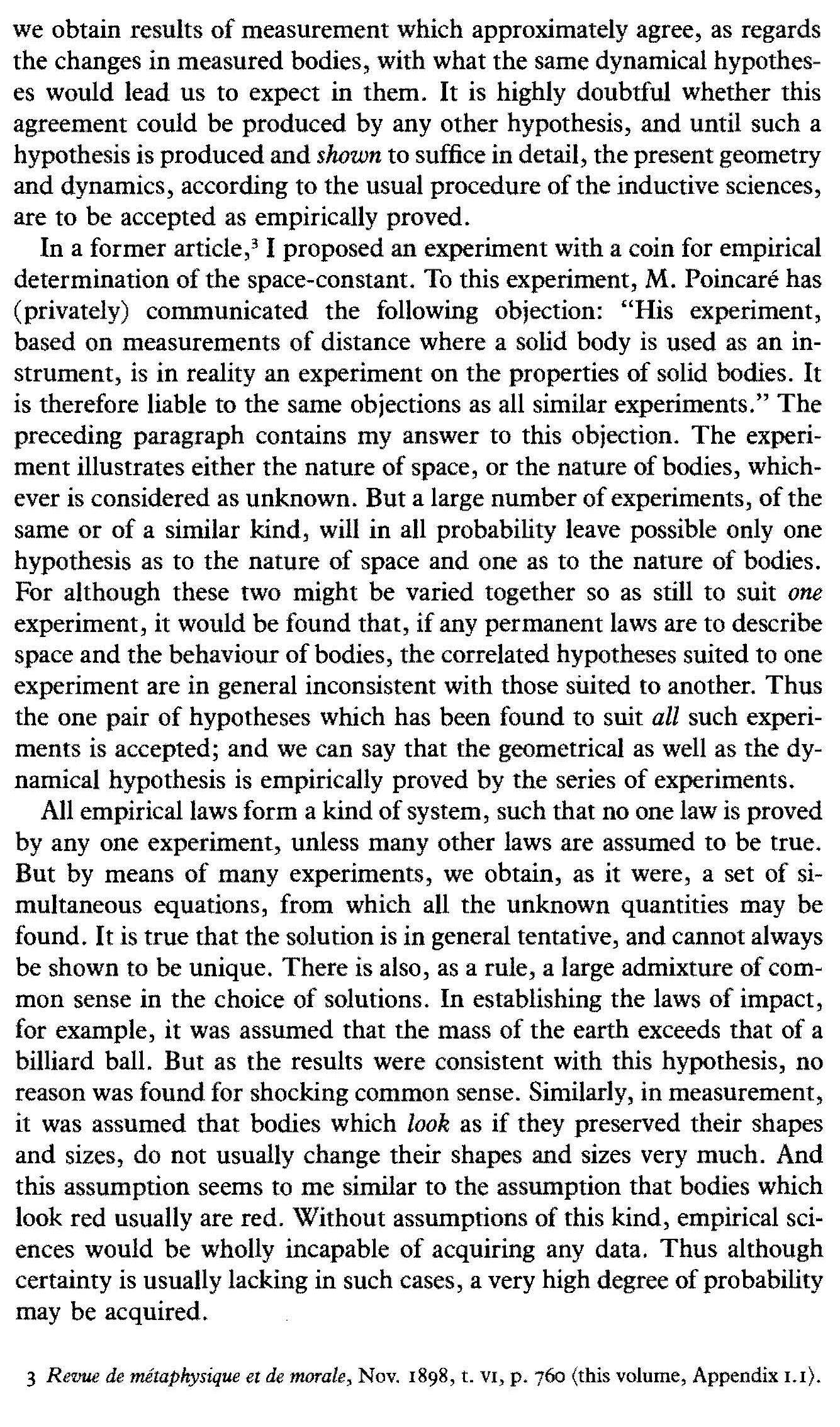The Axioms of Geometry 8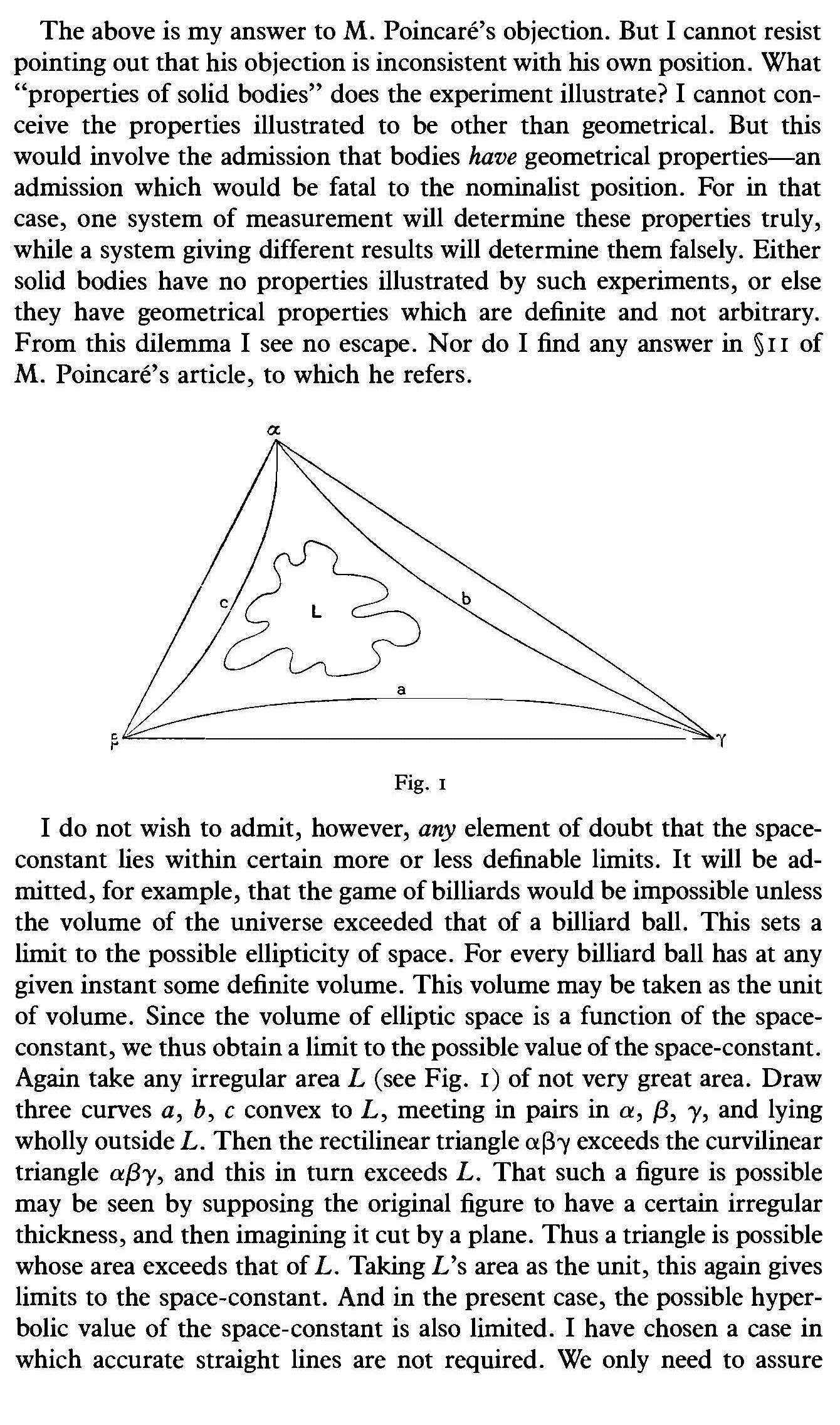The Axioms of Geometry 9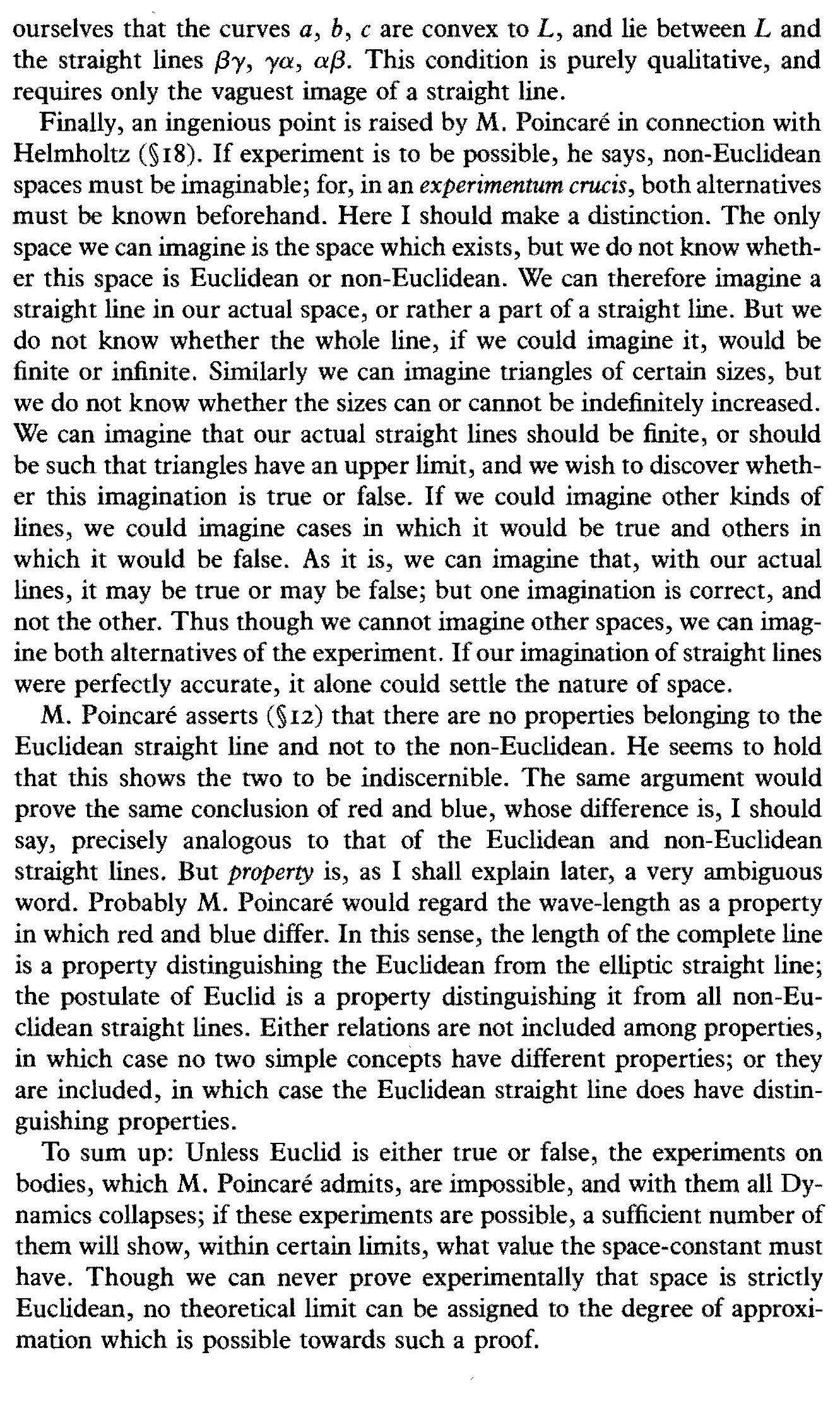The Axioms of Geometry 10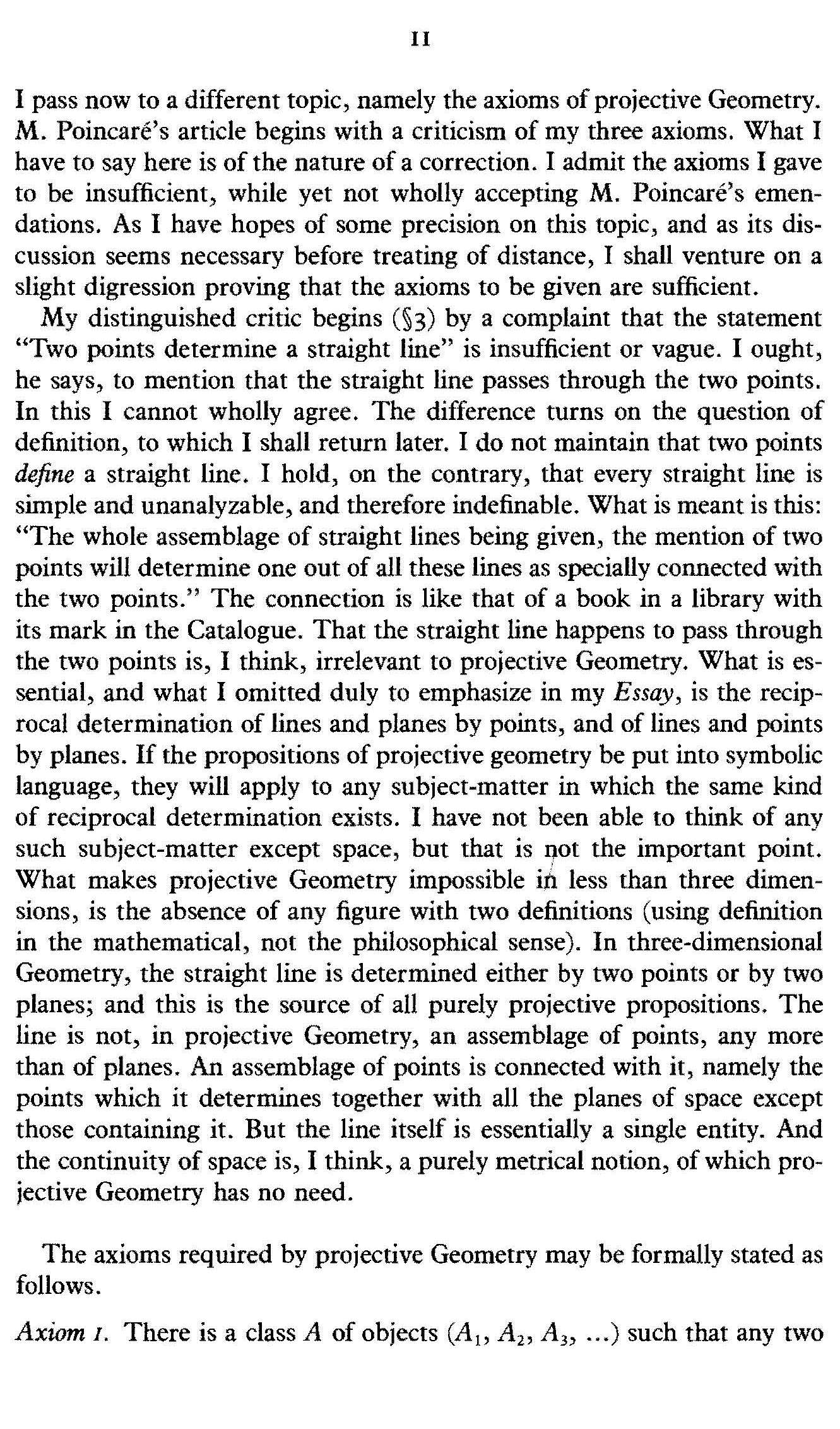The Axioms of Geometry 11The Axioms of Geometry 12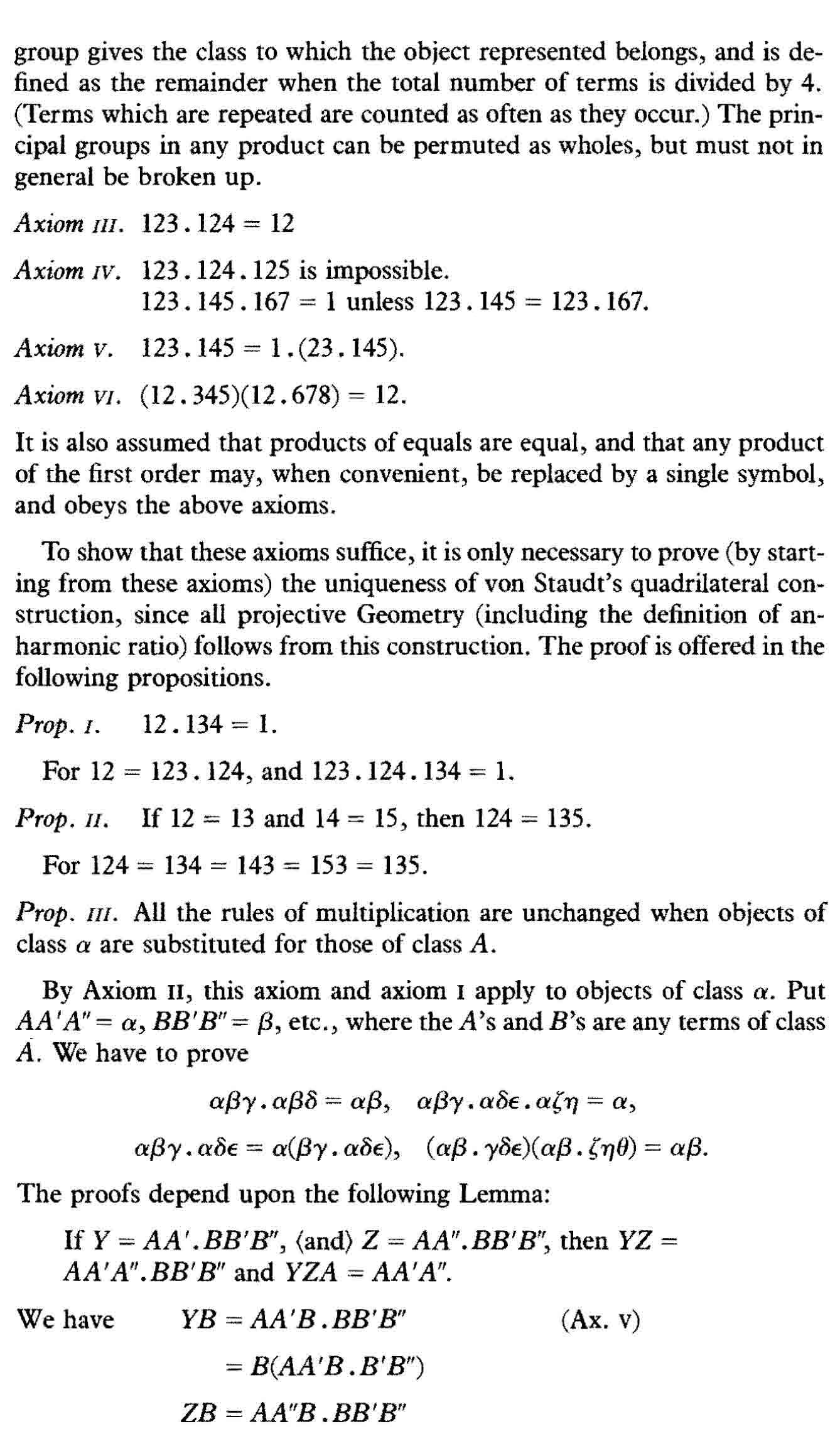The Axioms of Geometry 13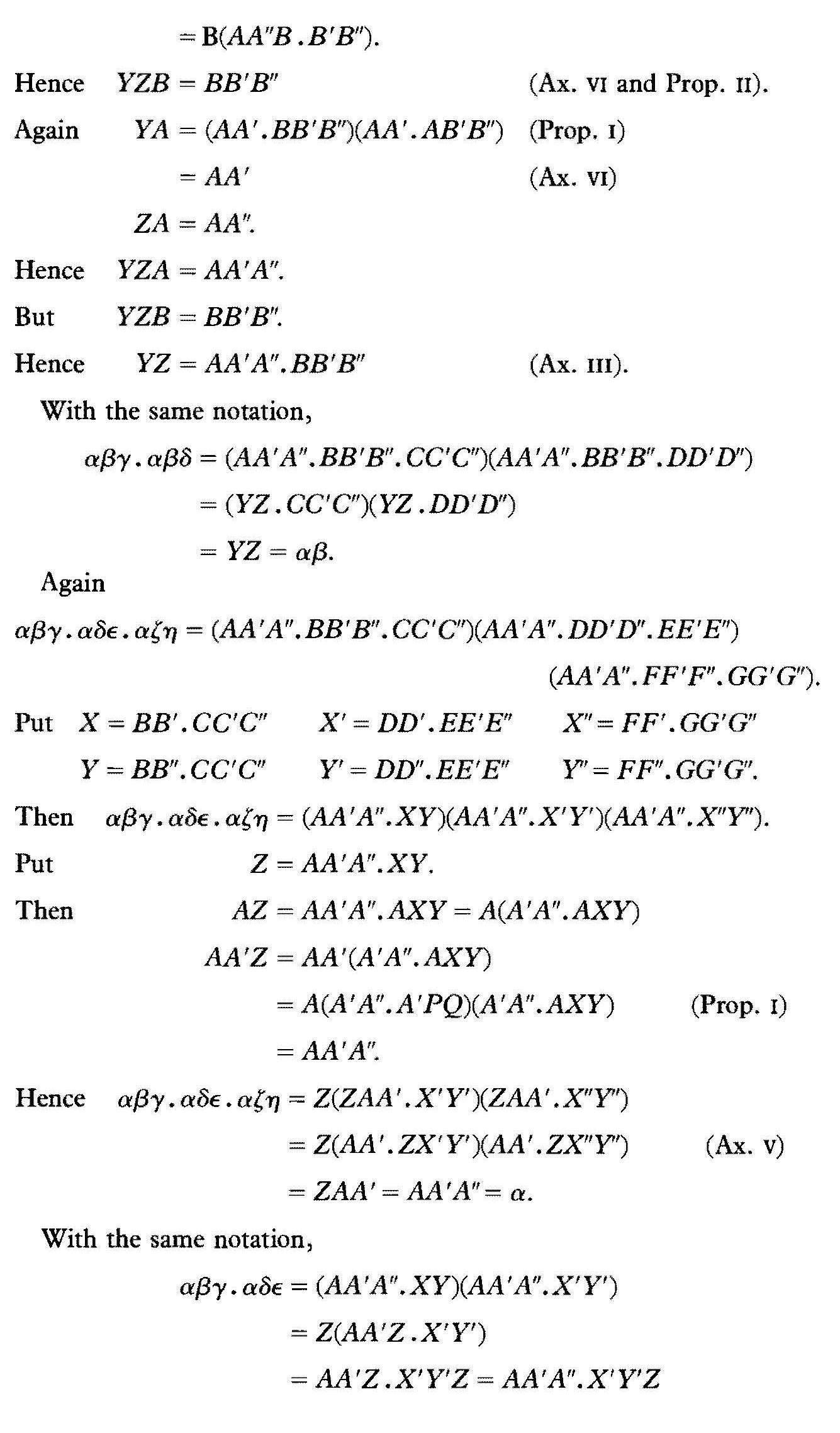The Axioms of Geometry 14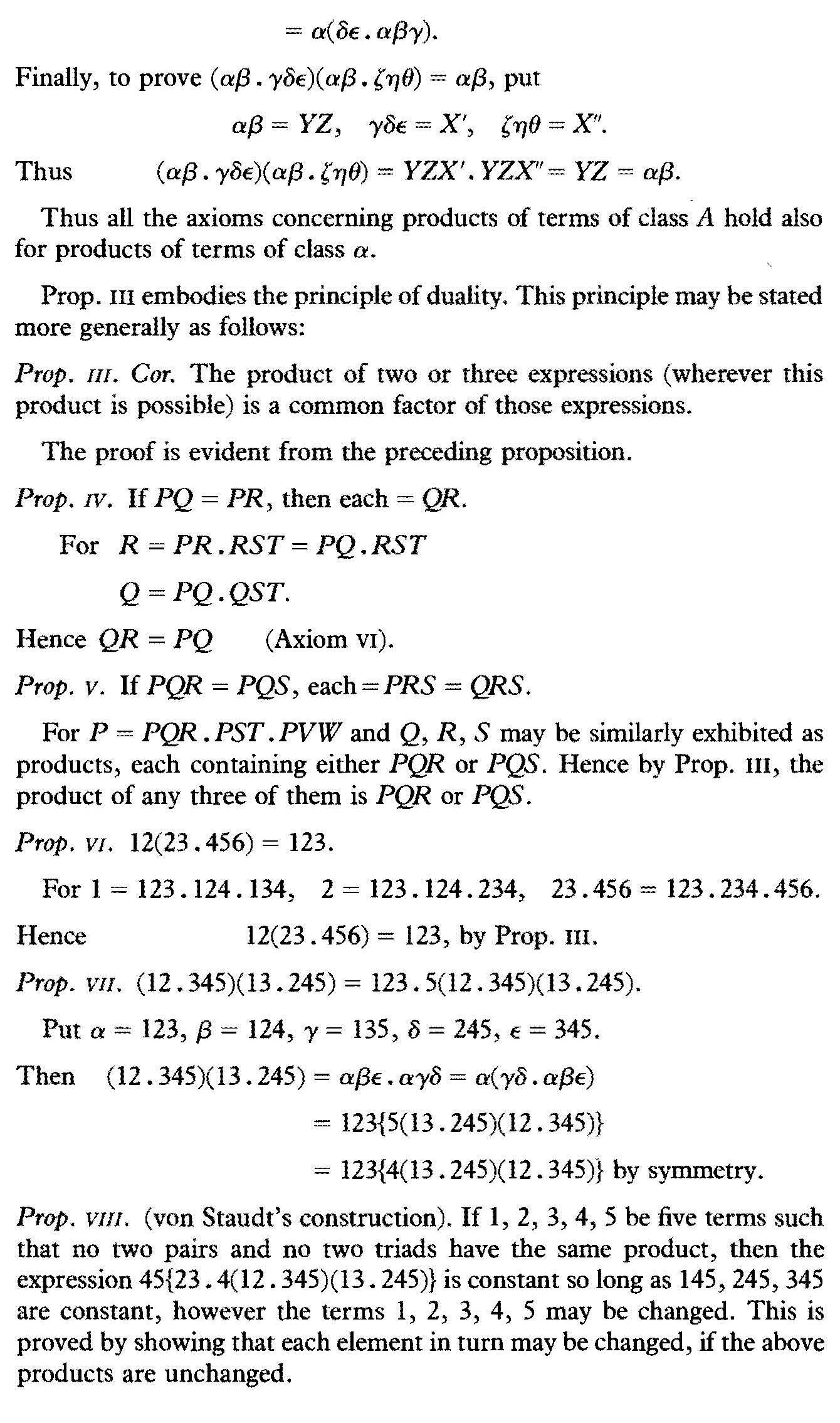The Axioms of Geometry 15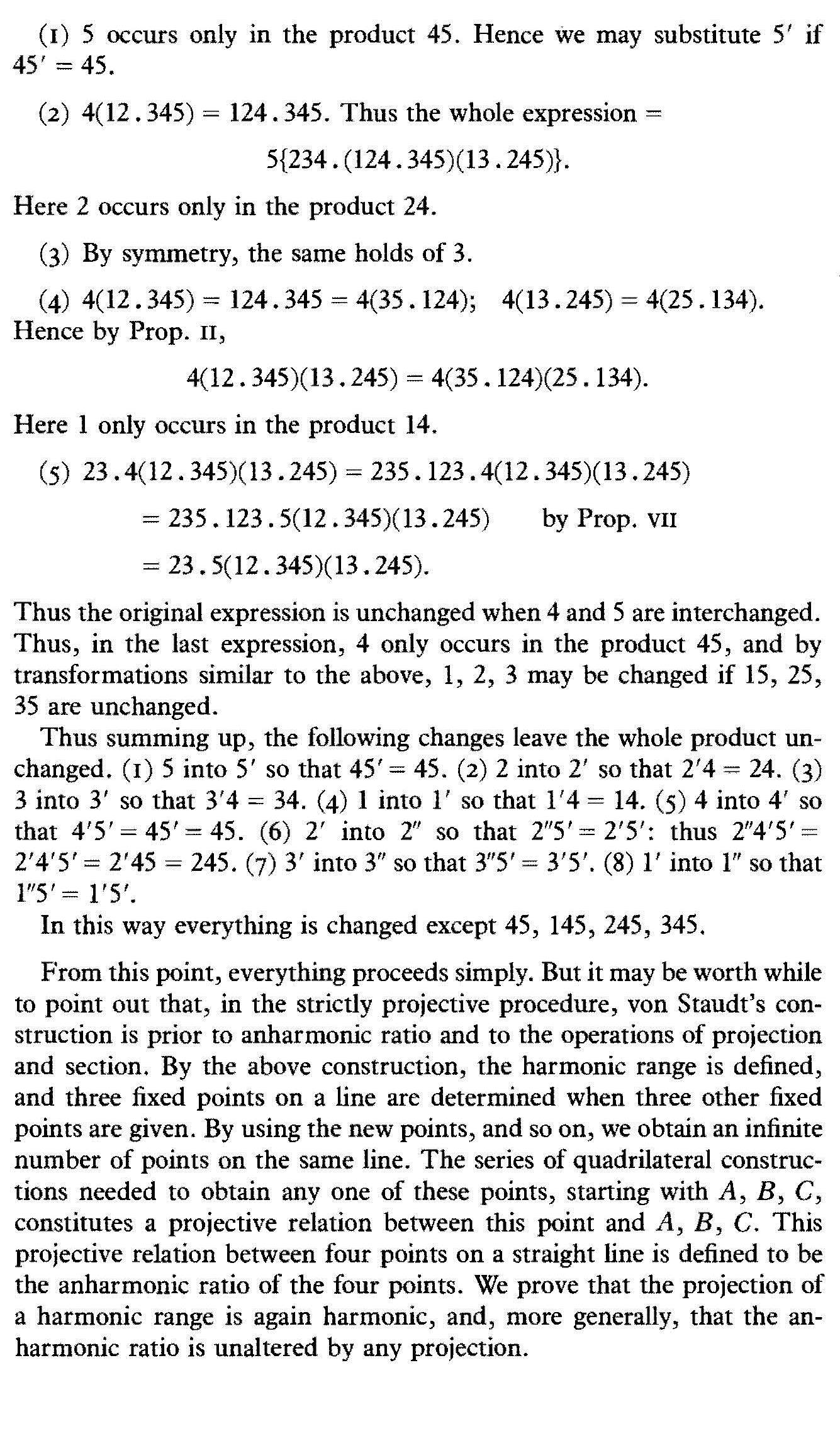The Axioms of Geometry 16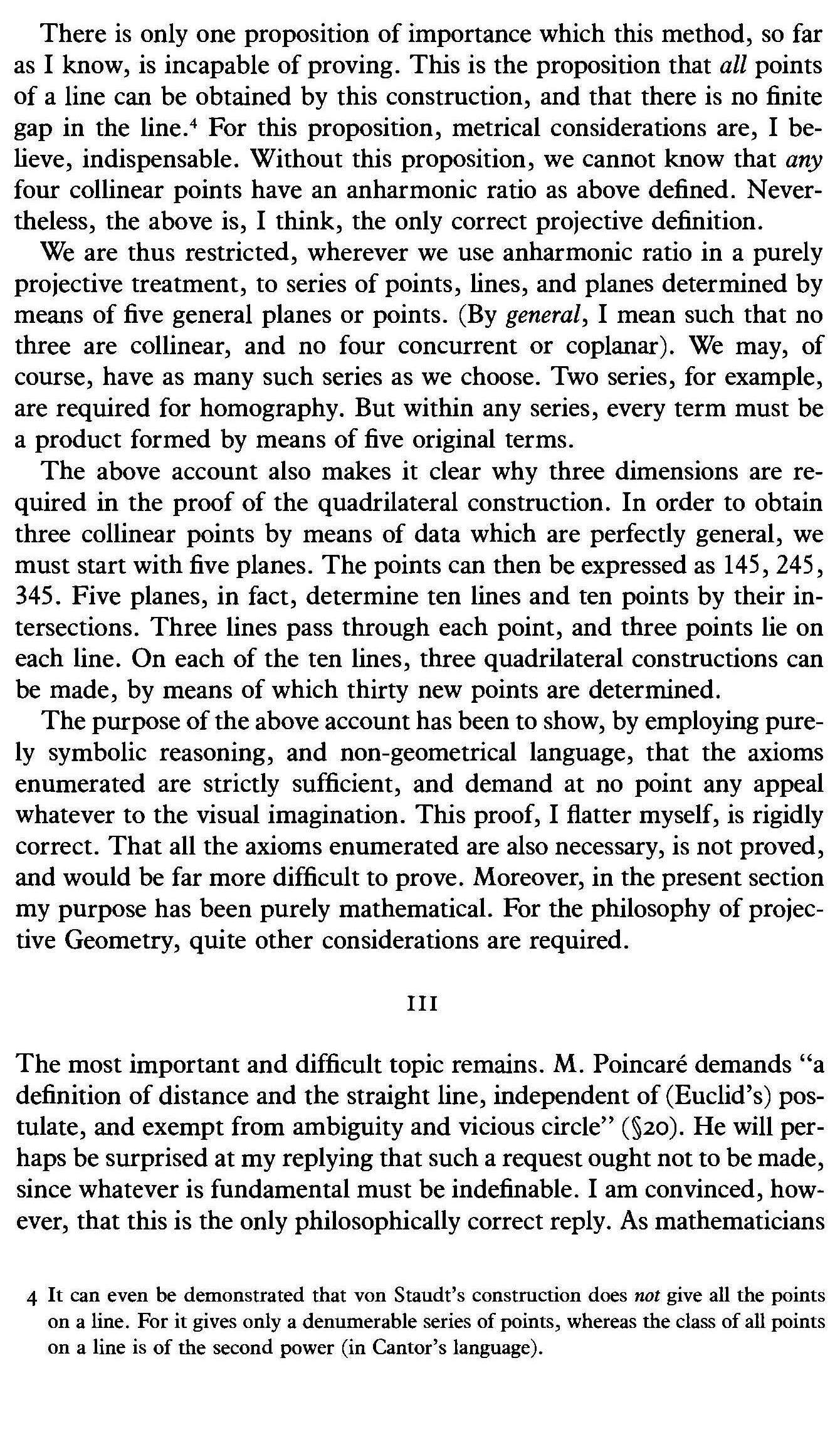The Axioms of Geometry 17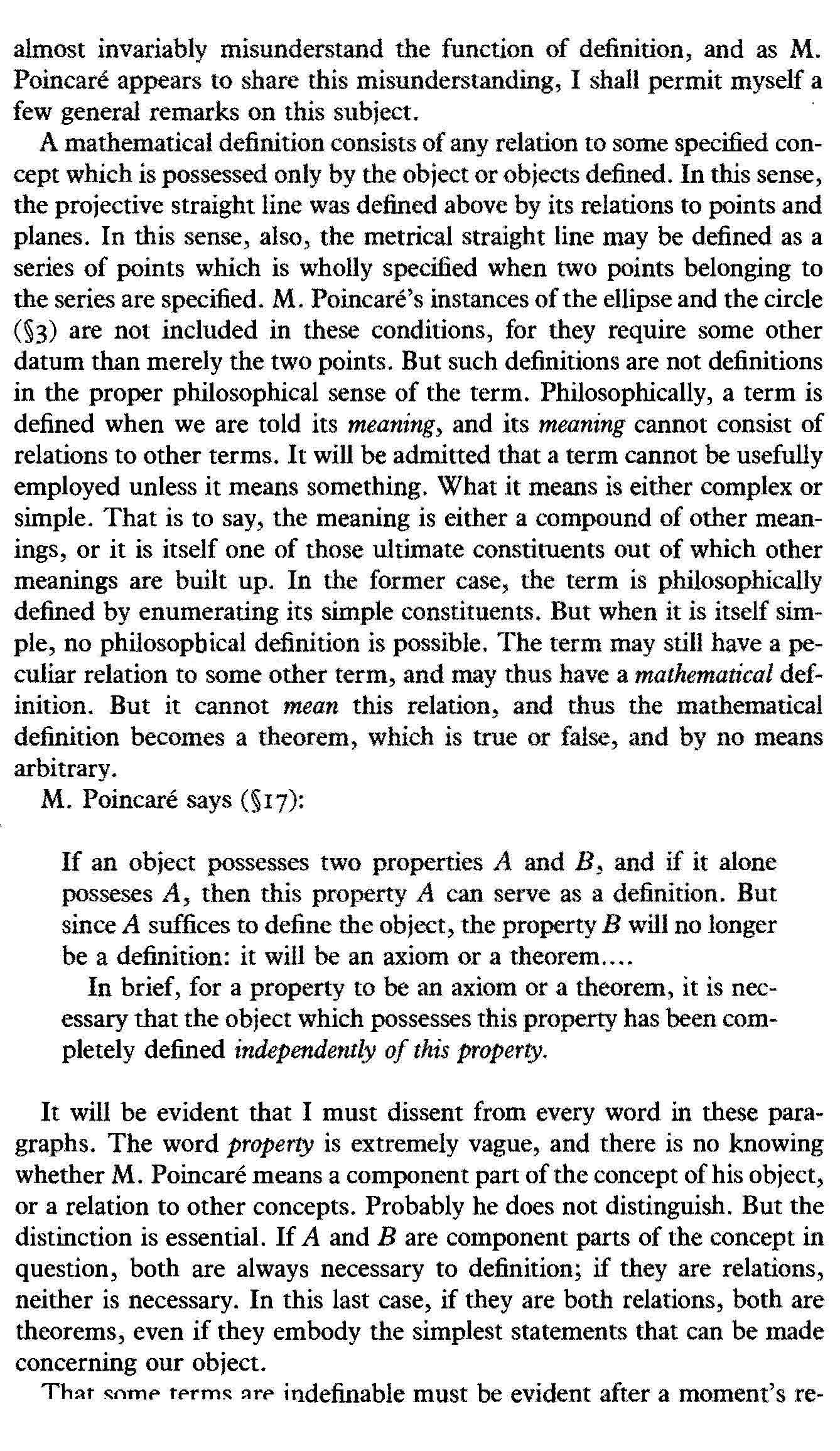The Axioms of Geometry 18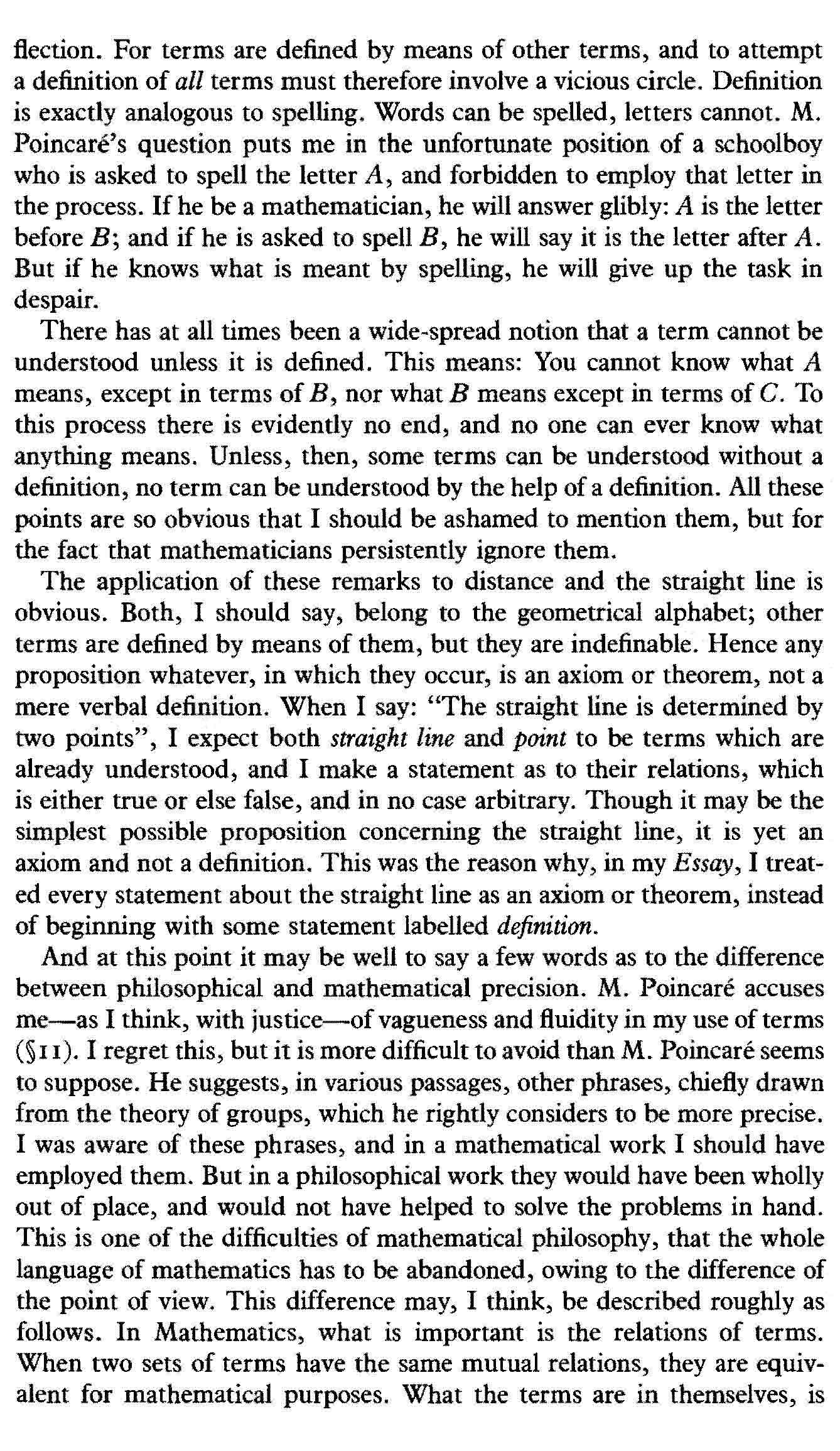The Axioms of Geometry 19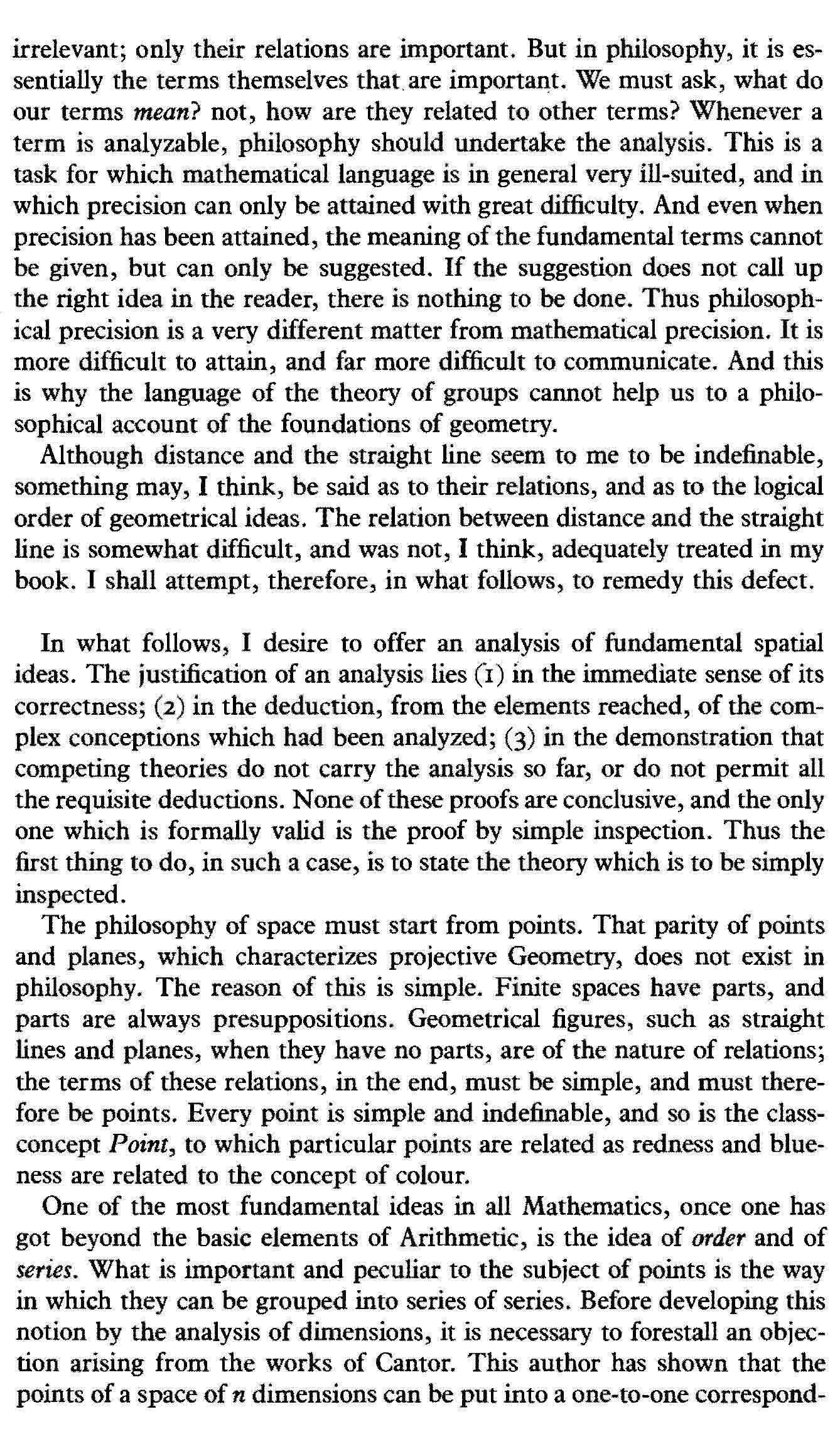The Axioms of Geometry 20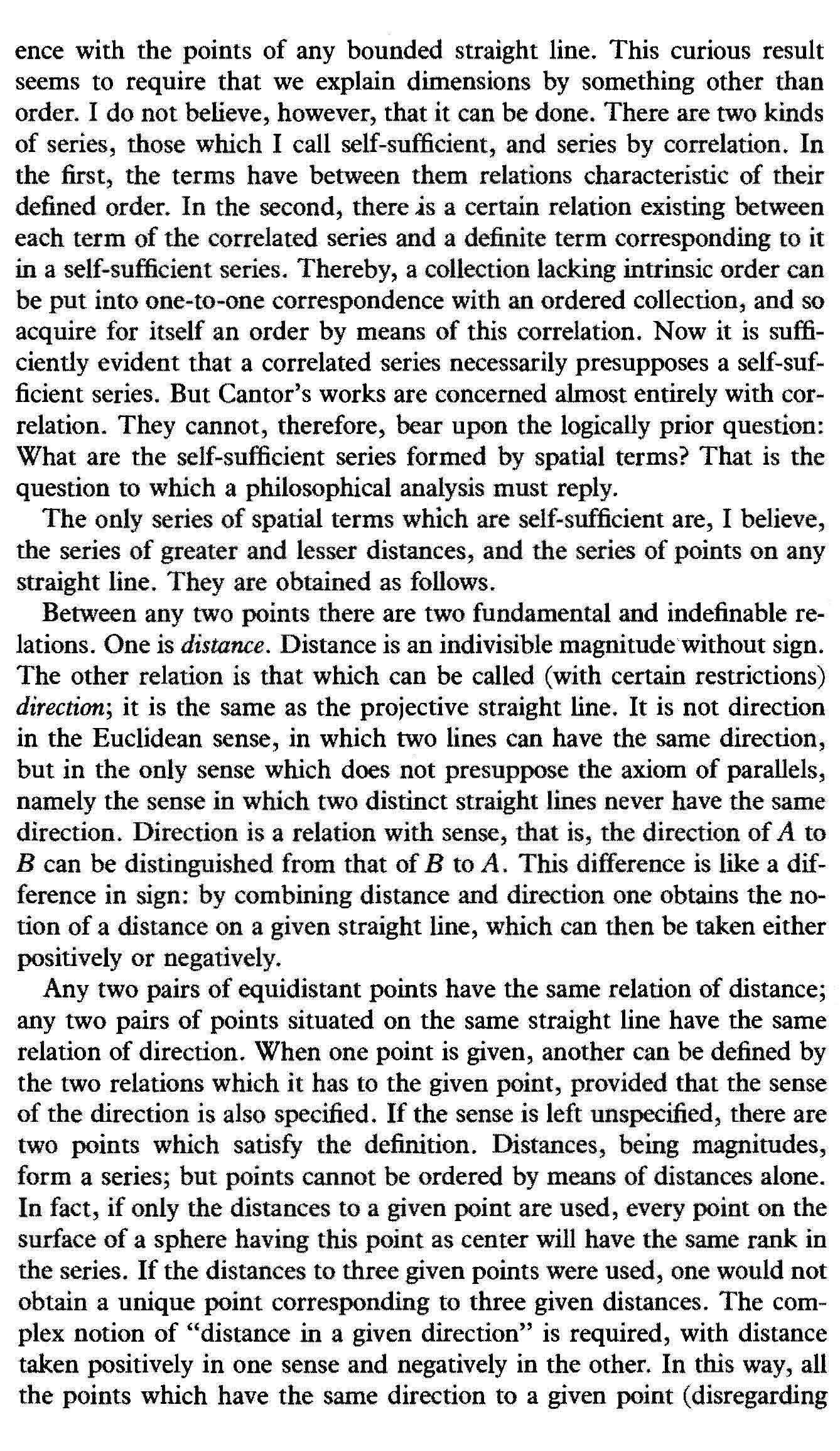The Axioms of Geometry 21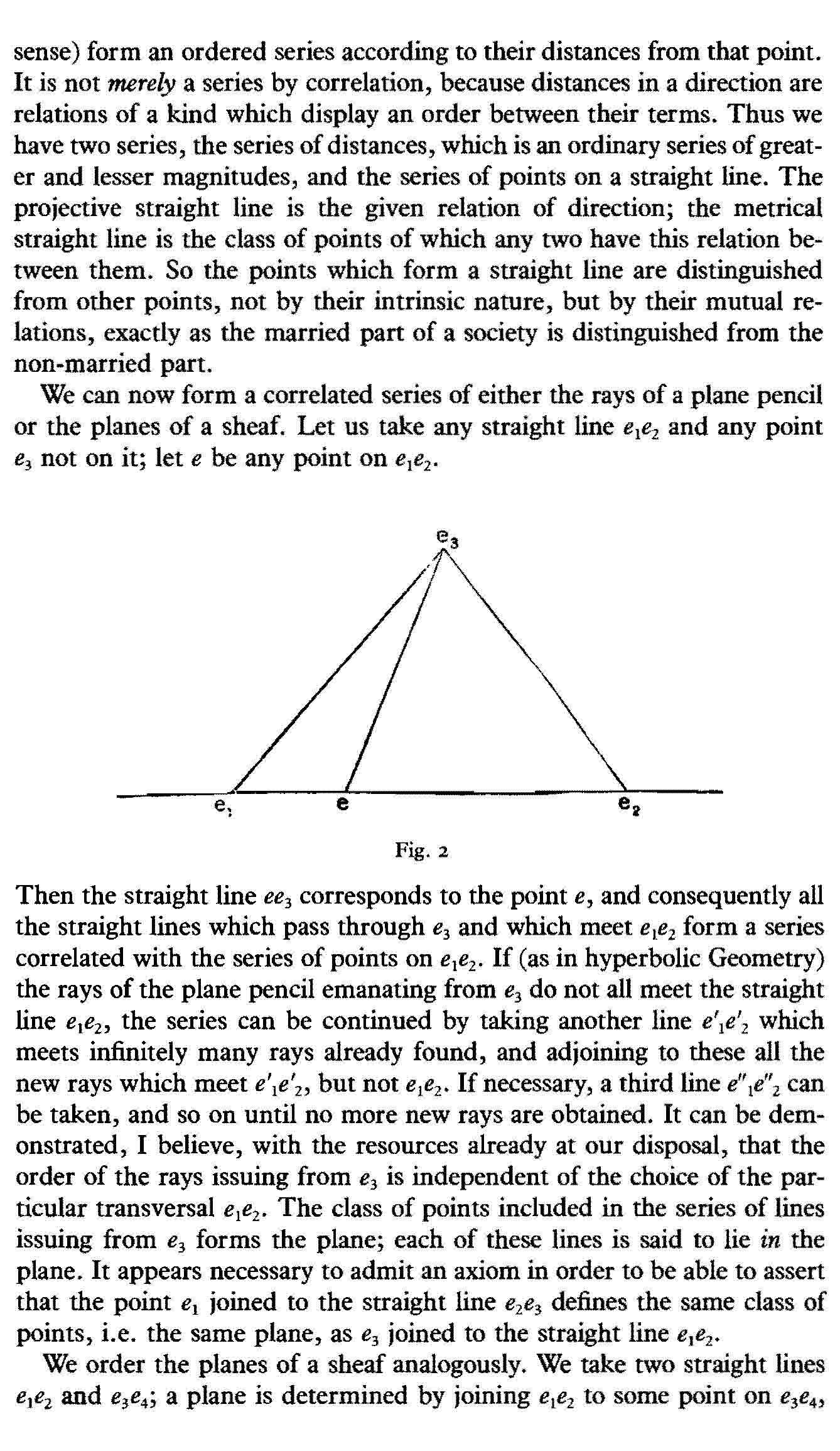The Axioms of Geometry 22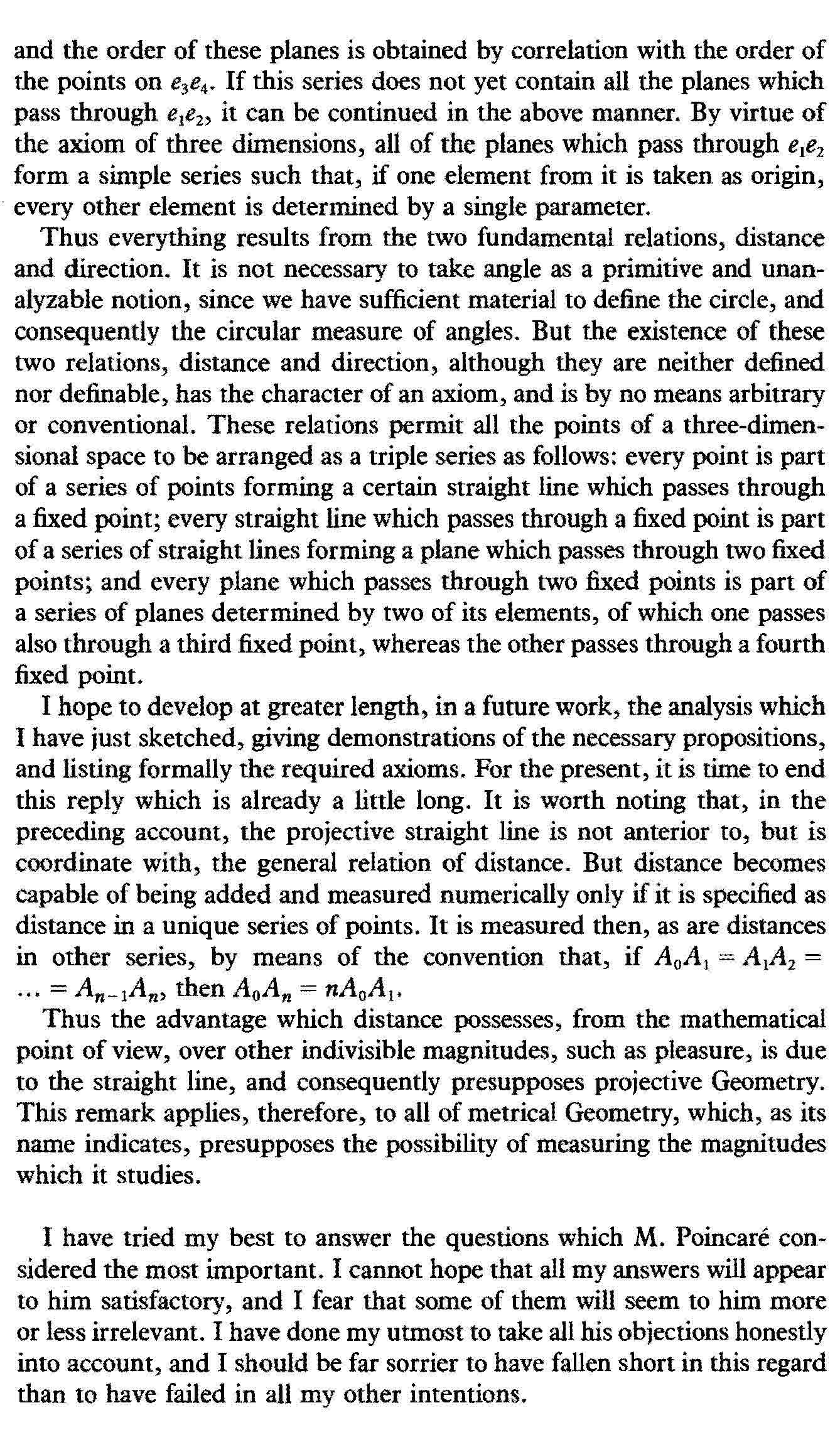*  Bertrand Russell, “The Axioms of Geometry,” Revue de Métaphysique et de morale 7 (Nov 1899), 684-707  Trans. Papers 2  Reply to Pioncaré’s review of Russell’s Essay on the Foundations of Geometry